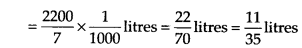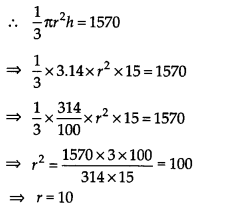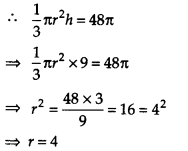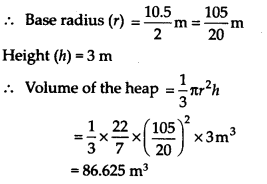NCERT Solutions for Class 9 Maths Chapter 13 Surface Areas and Volumes Ex 13.7

# NCERT Solutions for Class 9 Maths Chapter 13 Surface Areas and Volumes Ex 13.7

## NCERT Solutions for Class 9 Maths Chapter 13 Surface Areas and Volumes Ex 13.7

NCERT Solutions for Class 9 Maths Chapter 13 Surface Areas and Volumes Ex 13.7 are the part of NCERT Solutions for Class 9 Maths. Here you can find the NCERT Solutions for Chapter 13 Surface Areas and Volumes Ex 13.7.

## NCERT Solutions for Class 9 Maths Chapter 13 Surface Areas and Volumes Ex 13.7

Ex 13.7 Class 9 Maths Question 1.
Find the volume of the right circular cone with
(i) radius 6 cm, height 7 cm
(ii) radius 3.5 cm, height 12 cm

Solution:
(i) Here, radius of the cone (r) = 6 cm
Height of the cone (h) = 7 cm

Ex 13.7 Class 9 Maths Question 2.

Find the capacity in litres of a conical vessel with
(i) radius 7 cm, slant height 25 cm
(ii) height 12 cm, slant height 13 cm

Solution:
(i) Here, radius (r) = 7 cm and slant height (l) = 25 cmThus, the required capacity of the conical vessel is 1.232 litres.

(ii) Here, height (h) = 12 cm and slant height (l) = 13 cm

Capacity of the conical vesselThus, the required capacity of the conical vessel is 11/35 litres.

Ex 13.7 Class 9 Maths Question 3.
The height of a cone is 15 cm. If its volume is 1570 cm³, find the radius of the base. (Use π = 3.14)

Solution:
Here, height of the cone (h) = 15 cm
Volume of the cone = 1570 cm3
Let the radius of the base be ‘r’ cm.Thus, the required radius of the base is 10 cm.

Ex 13.7 Class 9 Maths Question 4.
If the volume of a right circular cone of height 9 cm is 48 cm³, find the diameter of its base.

Solution:
Volume of the cone = 48 cm3
Height of the cone (h) = 9 cm
Let the base radius be r cm.Diameter of the base of the cone = (2 × 4) cm = 8 cm

Ex 13.7 Class 9 Maths Question 5.
A conical pit of top diameter 3.5 m is 12 m deep. What is its capacity in kilolitres?

Solution:
Here, diameter of the conical pit = 3.5 m
Radius of the conical pit (r) = 3.5/2 m = 35/20 m,
Depth (h) = 12 m

Volume (capacity) = 1/3 × πr2hThus, the capacity of the conical pit is 38.5 kl.

Ex 13.7 Class 9 Maths Question 6.
The volume of a right circular cone is 9856 cm3. If the diameter of the base is 28 cm, find:
(i) height of the cone
(ii) slant height of the cone
(iii) curved surface area of the cone

Solution:
Volume of the cone = 9856 cm3
Diameter of the base = 28 cm
Radius of the base (r) = 28/2 = 14 cm

(i) Let the height of the cone be h cm.

Thus, the required height is 48 cm.

(ii) Let the slant height be l cm.
l2 = r2 + h2
l2 = 142 + 482 = 196 + 2304 = 2500
l = 50
Thus, the required slant height is 50 cm.

(iii) The curved surface area of a cone = πrl
Curved surface area = 22/7 × 14 × 50 cm2
= 2200 cm2
Thus, the curved surface area of the cone is 2200 cm2.

Ex 13.7 Class 9 Maths Question 7.
A right triangle ABC with sides 5 cm, 12 cm and 13 cm is revolved about the side 12 cm. Find the volume of the solid so obtained.

Solution:
Sides of the right triangle ABC are 5 cm, 12 cm and 13 cm.
The right-angled triangle is revolved about the side 12 cm.

Thus, we have radius of the base of the cone so formed (r) = 5 cm
Height (h) = 12 cm
Volume of the cone so obtained = 1/3 × πr2h
1/3 × π × (5)2 × 12 cm3
= 100 π cm3
Thus, the required volume of the cone is 100π cm3.

Ex 13.7 Class 9 Maths Question 8.
If the triangle ABC in Question 7 above is revolved around the side 5 cm, then find the volume of the solid so obtained. Find also the ratio of the volumes of the two solids obtained in Questions 7 and 8.

Solution:
Since the right triangle is revolved around the side 5 cm.
Height of the cone so obtained (h) = 5 cm
Radius of the cone (r) = 12 cm

Thus, the required ratio is 5 : 12.

Ex 13.7 Class 9 Maths Question 9.
A heap of wheat is in the form of a cone whose diameter is 10.5 m and height is 3 m. Find its volume. The heap is to be covered by canvas to protect it from rain. Find the area of the canvas required.

Solution:
Here, the heap of wheat is in the shape of a cone with base diameter = 10.5 mThus, the required volume = 86.625 m3

Now, the area of the canvas to cover the heap must be equal to the curved surface area of the conical heap.

Thus, the required area of the canvas is 99.825 m2.

You can also like these:

NCERT Solutions for Maths Class 10

NCERT Solutions for Maths Class 12